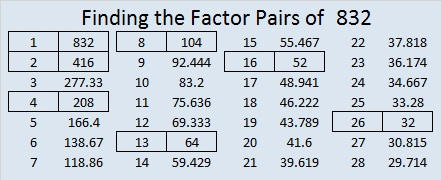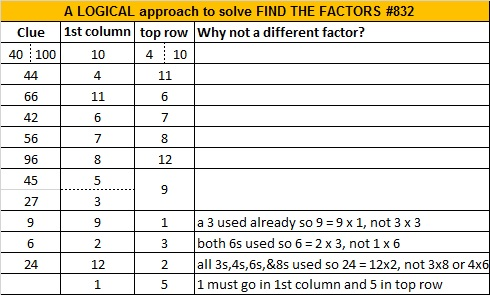# Facts, Factors, and a Countdown to 2023

### Countdown to 2023:

The last 10 seconds of the year, we like to countdown from 10 to the new year. I like a mathematical way of counting down so I try to make an equation with the numbers from 10 to 1 that equals the coming year. This year I could have based my countdown  on last year’s countdown and said
(10-9+8×7×6)(5-4)(3)(2)+1 = 2023,
but this is a blog about factoring so I want a countdown that takes you to the prime factors of 2023 first. Here’s my countdown: (Note: Even though I used 1 as a factor twice in the countdown, I am very much aware that 1 is not a prime factor of any number.)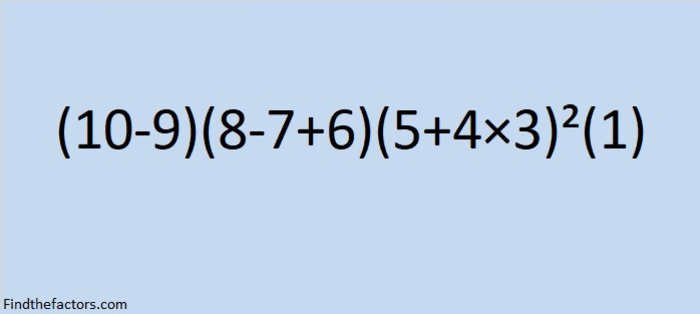make science GIFs like this at MakeaGif
Here’s the final shot from the Countdown: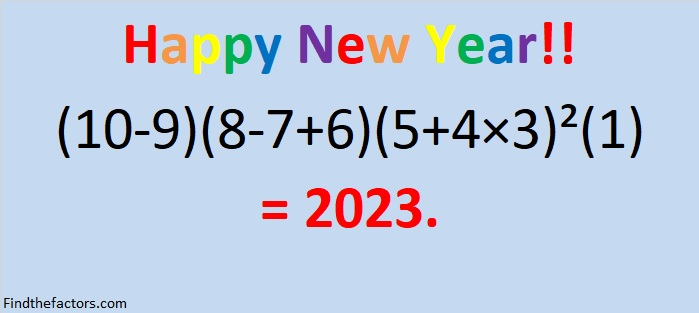### A Puzzle for 2023:

2023 has something in common with 2022. When either number and their reverses are squared, something interesting happens…it’s almost like looking in a mirror!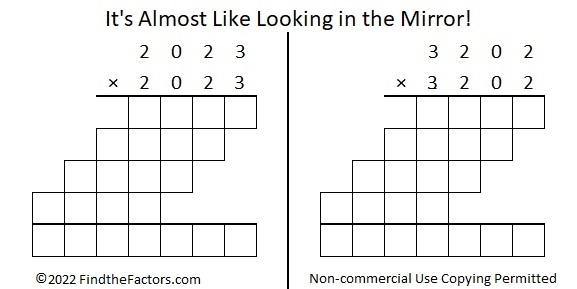Only 50 numbers less than 10000 can make a similar claim to fame:### Factors of 2023:

• 2023 is a composite number.
• Prime factorization: 2023 = 7 × 17 × 17, which can be written 2023 = 7 × 17².
• 2023 has at least one exponent greater than 1 in its prime factorization so √2023 can be simplified. Taking the factor pair from the factor pair table below with the largest square number factor, we get √2023 = (√289)(√7) = 17√7.
• The exponents in the prime factorization are 1 and 2. Adding one to each exponent and multiplying we get (1 + 1)(2 + 1) = 2 × 3 = 6. Therefore 2023 has exactly 6 factors.
• The factors of 2023 are outlined with their factor pair partners in the graphic below.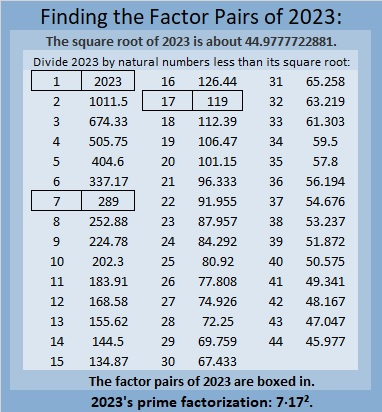### More About the Number 2023:

What do 2023 tiny squares look like?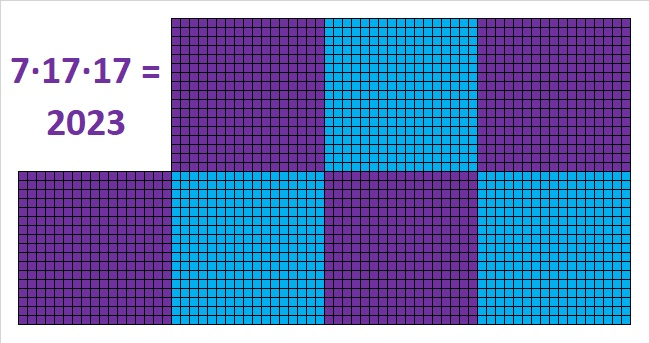2023 is the sum of consecutive numbers in five different ways: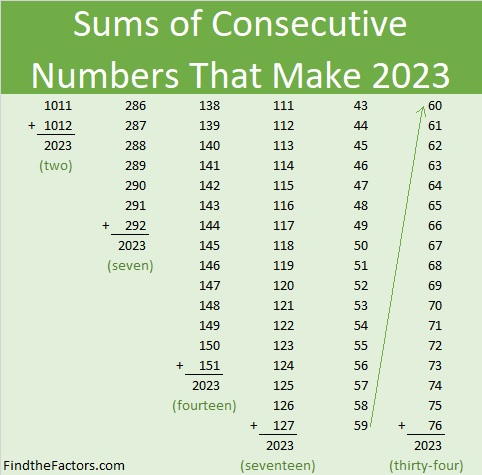And it is the sum of consecutive odd numbers in two ways: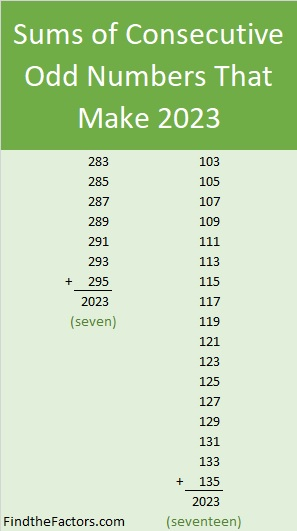2023 is a palindrome in base 16 because
7(16²) + 14(16) + 7(1) = 2023.

This tweet demonstrates that the prime factors of 2023 have a relationship with the digits of 2023.

I saw this tweet on Twitter and immediately recognized something else cool about 2023.

That might seem like a lot of mathematical mumble jumble, but with a little bit of explanation, it can be understood. And even though I made the problem look scarier because I substituted 2+0+2+3 for 7, some older elementary students who already understand powers, factorials, and/or remainders, will get it. I’m confident you can too.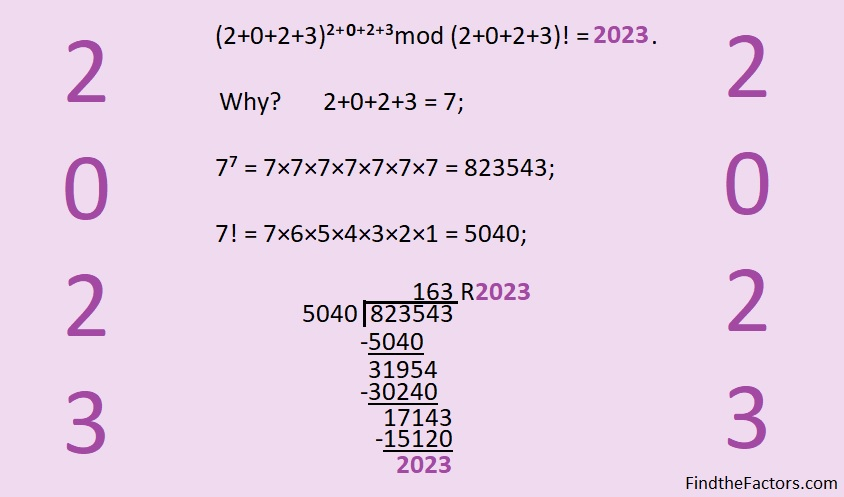You could also give the following list of facts to older elementary students and ask them to use it to find the remainder when they divide 823,543 by 5040.

1×5040 =    5040
2×5040 = 10080
3×5040 = 15120
4×5040 = 20160
5×5040 = 25200
6×5040 = 30240
7×5040 = 35280
8×5040 = 40320
9×5040 = 45360

Because its factors, 17 and 289, are hypotenuses of Pythagorean triples, 2023 is also the hypotenuse of some Pythagorean triples:
952-1785-2023 which is 119(8-15-17) , and
1127-1680-2023 which is 7(161-240-289).

Ureczky József also pointed out in the comments of this post, that 2023 is the short leg in SIX Pythagorean triples, and thus
2023² = 2046265² – 2046264²
2023²= 292327² – 292320²
2023² = 120377² – 120360²
2023² = 17255² – 17136²
2023² = 41785² – 41736²
2023² = 7225² – 6936²

One of those triples is a primitive triple. Can you determine which one?

Ureczky József shared one more amazing fact in the comments that I’m replicating here:

2023=9³+8³+7³+6³+5³+4³+3³+2³-1³

### More Mathematical Tweets About 2023:

These tweets are more or less in the order I saw them, not in order of mathematical difficulty. I will add more as I see them.

v

# 127th Playful Math Education Blog Carnival

Ladies and gentlemen welcome to the Playful Math Education Blog Carnival featuring the incredible number 127 of the famous Mersenne Prime family! Let’s give a big hand to. . . . .

2²-1 = 3, the smallest single Mersenne Prime;
2³-1 = 7, the smallest double Mersenne Prime;
2⁷-1 = 127, the smallest triple Mersenne Prime; and finally
39-digit 2¹²⁷-1, the smallest quadruple Mersenne Prime!

It took NINETEEN YEARS (1857 to 1876) for Édouard Lucas to test Mersenne Prime Number 2¹²⁷-1 BY HAND to successfully verify that it is indeed a prime number. It is the largest Mersenne Prime that has ever been verified by hand calculations!

Ladies and gentlemen, Prime number 127 has one more amazing feat up its sleeve that you will have to see to believe:In this month’s blog carnival posts, the amazing Desmos will delight and astound young and old alike. There will even be an Easter egg hunt! The blog post links (in turquoise) are joined by several links from Twitter (in blue-violet) and a few from other places such as Youtube (in red). Stay as long as you like and ENJOY what the many carnival participates have to offer in 20 different amusement areas!

Art and Mathematics

1. Stephanie showed off her colorful and impressive Tessellation Math Art Wall on twitter.
2. David Petro used all 84 pieces of a 21st-century pattern block set to create a lovely symmetrical design.
3. After this year’s very long winter, I’m especially glad Colleen Young collected some lovely and amazing springtime Desmos drawings and gifs in It’s Springtime. . . .
4. Do you see mathematics everywhere? Continuous-Everywhere-but-Differentiable-Nowhere can and does, even on a student’s shirt. Read the story and see the t-shirt design replication in Desmos in Going Off the Beaten Path.
5. Wanting to have your students create an art project in Desmos? 1ntegration-by-Parts has given that assignment many times and has links for student directions to help them meet your expectations in Desmos Art Project (Update).
6. You must click on the turtle face link in Desmos Art! With just ONE equation, a magnificently detailed turtle face was produced in the Desmos calculator. I was so impressed. I tweaked that single equation by changing the number 16 to 7.29, added some color restrictions as well as equations to make a mouth and some eyes to produce my own Desmos Art piece I call Blue-eyed Beauty.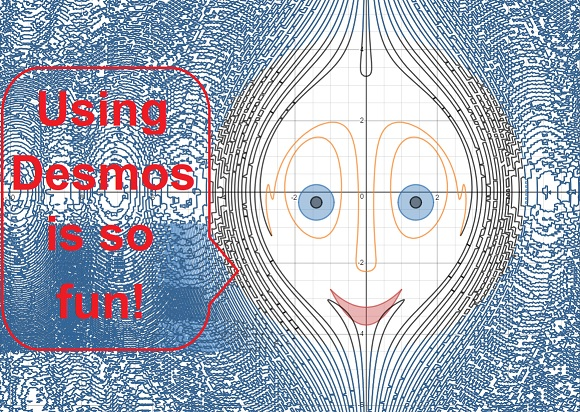Classwork/Homework that is enjoyable

1. Elementary-school-age students will enjoy that Matt Vaudrey shared.
2. I-Speak-Math has a mathematics homework solution students LOVE. Read about it in Meaningful Homework and CPM.
3. Jennifer Michaelailis has a pro tip on how to keep students who need a little extra help in class from feeling defeated.
4. If you want a free math education gathering in your area, here’s how to get one started. Also, check out Denise Gaskins’s resources to keep the group going.

Creative Writing

1. Many people have a personal story to tell that explains why they love mathematics. Through a Mathematician’s Eyes opens up and shares her experiences in My Story. What obstacles did she face? How did she feel about enjoying a subject so many others hated?
2. Philip Jose Pacis played with some math vocabulary and wrote a poem he titled Fractions about a fractured relationship.
3. Calendars and time are mathematical topics. How many other mathematical terms do you see in Maggie C.’s poem On Time? Do any of those words have more than one meaning?

Decimals

1. Math Geek Mama has come up with Decimals on a Number Line Game and includes everything you need to teach this concept with complete confidence.
2. Would you like to play Decimal Pickle? On Twitter, Mrs. Unger explained how to play it as well as a few of its variations.

Desmos First Aid Station?

1. There’s no need to call the paramedics when it’s time to learn about parametric functions. Suzanne Van Oy has come to the rescue! She sees a lot of value in parametric functions and their graphs. Why is she so excited about them? What’s all the fuss? Suzanne answers those questions and more in Why Parametrics?.
2. Suzanne Van Oy also recently put an incredible spinning Desmos Birthday Cake on twitter. How does she make Desmos gifs that don’t look like they need to be put on life support? Six months ago she blogged about how to do it in Making a Great Desmos Gif. She certainly knows what she’s doing!
3. Sometimes Desmos doesn’t do what you expect. Your work might need some first aid. DesmosGraph (Unofficial)’s post: Desmos Traps: Why Is It Not Working may have the diagnosis and cure you seek.
4. Although this post from Gold & Ratios is two years old, I still wanted it included on this list. It teaches how to add color and texture to Desmos.

Eggstraordinary Mathematical Easter Egg Hunt from Twitter

1. Cliff Pickover shared Don M. Jacobs equation for an egg laying on its side.
2. Tamás Görbe shared an equation for an egg that is standing up on its wide end.
3. Get out your markers and start coloring Paula Beardell Krieg’s and Three eggs! Paula also shows how
4. Mathigon shared a gif of a colorful, nine-piece, tangram-like egg puzzle that can also transform into a rabbit and a goose.
5. Robert Bosch shared that have a few mathematical traits in common.
6. Ha! Doesn’t this always happen? I found more fabulous eggs the day after the hunt: Daniel Mentrard’s eggs made in Geogebra,
7. And these Eggsponenential eggs created by Traci Jackson!

Exponents and Exponential Functions

1. Christopher Danielson on Twitter that generated a lot of thinking from adults. I’m sure it would do the same for kids who understand a little bit about exponents.
2. Jongarland6 was able to get ELL students conversing with each other in English about exponential functions. How it was done is described in Desmos Sparking Academic Conversations.

Fractions

1. Math Play Day gives ideas from 20SomethingKids and 1KookyTeacher about playful ways first-grade kids learned about fractions.
2. Mathgeek Mama published some adorable free Equivalent Fraction Robot Puzzles.
3. A recently released YouTube video has a little girl teaching about fractions in Maths 4 Kids’ Fractions of Shapes and Fraction Vocabulary.
4. Here’s another one featuring the same little girl: Fractions of Amounts Using the Bar Model
5. Every carnival has food concessions. You can have a lesson at breakfast on fractions based on CTSPEDMATHDUDE’s post Sausage Fractions: Real Life Example.
6. When teaching fraction division, should you start with rules or diagrams? Filling the Pail speaks from experience in Fraction Division and explains the advantages and disadvantages of both approaches.

Games and Educational Toys1. On the spur of the moment, I came up with a very quick Yahtzee variation that I played with one of my students. We counted the number of rolls it took us to get a Yahtzee. Lowest score wins. He beat me badly every round as the graphic above this category attests, but on a different day, Lady Luck was with me more than him.
2. Denise Gaskin also has a tried and true Yahtzee game variation that she calls Six Hundred. You only need to print her directions and scoring sheets, provide six 6-sided die and pen or pencils, and you’ll be ready to make memories in more ways than one.
3. Autism Awareness Week was earlier in April. In this post, Special Educational Resources Blog reviewed three games made by Orchard Toys: Money Match Cafe (teaches about money), Look and Find Jigsaws (teaches number and letter recognition), and Bus Stop (uses processes like 3-2+4-1 to figure out how many kids are on the bus when the bus arrives at the bus station.)
4. The Mathematical Tourist shared how to play a game called Clobber. The game has been around since 2001, but the best strategy to win the game is still a mystery. I’m sure getting clobbered will be just as much fun as winning.

Geometry

1. What did an insightful five-year-old tell Paula Beardell Krieg about triangles?
2. Simon Gregg showed some pictures demonstrating how students had fun exploring squares in several different ways.
3. Similar geometric shapes line up and beg you to compare their dilations in Paula Beardell Krieg’s post About Halfway There.
4. Robert Loves Pi creates wonderful 3-D rotating digital geometric models. This one he calls the Twelfth Stellation of the Triakis Tetrahedron.
5. Desmos is a great tool, but sometimes I need to actually see how people use it to teach. Bearsemath.com does exactly that by sharing some pictures of Desmos Geometry being presented to a class of 10th graders.

Giving Back

1. When Women Inspire gives Three Worthy Reasons to Teach Charity to Your Kids. One of those reasons is that they will naturally learn the mathematics of money management.
2. Read the impressive CBS News account of how a STEM Robotics team made a toddler wheelchair for a  two-year-old whose family couldn’t afford one.
3. LMS Life Skills was practically speechless! Her class designed quilts blocks by using linear equations. Then the class made two quilts and donated them!

Linear Equations

1. Wheeler’s Thoughts on Teaching used a bank balance problem to teach about solving a system of linear equations. The students were able to think about the problem and work on it with much fewer hints from their teacher. That makes teaching math much more fun for the students as well as the teacher.
2. Jeff Lay created an Easter egg hunt activity to review linear equations, and he is happy to share the google docs he made with you.
3. Ms. Wheeler exclaimed that sometimes crayons and paper do the trick while her glass created stain class art.
4. Coincidentally, Ian Maclellan also had his class produce some stain glass art with linear equations.
5. Alicia Phillips shared one of her student’s projects that used only linear equations and was made on Desmos.

Literature and Mathematics

1. Imagine this carnival ride: a catapult that will send you flying through the air! Lana Pavlova and Meredith Wilkes have assisted Math Book Magic in creating the perfect design of an unforgettable carnival ride in Play with Your Math with Little Pea. How far will this catapult take you?
2. Erikson Institute writes how Anno’s Flea Market by Mitsumasa Anno, Which Would You Rather Be? By Willaim Steig, and Whose Shoes? By Stephen R. Swinburne are Three Books That Encourage Simple Graph Explorations with Young Ones.
3. Life Through a Mathematicians Eyes loves to curl up with a good book that features mathematics. Find out which books she has gathered and plans to read in her MathReadathon.
4. Kelly Anne Garner received several must-have mathematics in literature book suggestions from Twitter to build a fabulous math library. Check out the whole thread.

Museum of Mathematics

1. Chirag Mittal took charge of April’s birthday celebration of Leonard Euler. Did you know that Euler is credited with being the first to use letters from our alphabet and the Greek’s alphabet to represent some very important functions and numbers: Σ, f(x), e, i,  and π?
2. Alan Paar of Established 1962 has put together a tour of Wendover School and the way teachers taught and students there learned several mathematical topics and other subjects from 1868 to 1930.
3. Jo Morgan retweeted a tweet that caught my eye and was, therefore, the catalyst for bringing  MathigonOrg’s expansive and interactiveTimeline of Mathematics to this month’s carnival.

Number Theory
1. The number 127 is a centered hexagonal number as demonstrated by the graphic above.
2. Ramblings of a Writer recently marveled about how many things come in fours in her post Exploring the Number Four.
3. Dr. Helen J. Williams has pictures from a very playful session on “Fiveness”.
4. Science Switch had a few things to write about Belphegor’s Prime number, 1000000000000066600000000000001, in The Most Evil Number.

Optical Illusions

1. When there was a day off from school in the middle of the week, BMore Energy found plenty of kid’s activities in Manhattan’s Museum of Illusions.
2. Love Travelling takes us on a trip to see the fun-filled Vilnius Museum of Illusions. There is so much to see there!
3. While Matematickcom shows how to make a paper optical illusion that you can make yourself in very little time.

Puzzles

1. Math with P. Nik gives instructions and several examples of his Three Elastic Bands puzzles. He said they were easy to make, so I made the one at the top of this category. Follow P. Nik’s instructions and you probably won’t need to click on the tiny answer key under the puzzle.
2. When Simona Prilogan of Fiat Lux writes a number puzzle, it is much more than it appears.  You have to study patterns inside the puzzles to figure out what the relationship really is. Give her Wednesday Math Puzzle a try!
3. Simona included a bonus, information about Bolsover Castle, in her Monday Math Puzzle. You will find two different Math Puzzles in the middle of reading about the Castle!
4. This clever tie matching exercise from Math with P. Nik feels more like a puzzle than a worksheet. Can you match the graph families with the correct equation families?

Statistics and Probability

1. Yes, you can do statistics in Desmos! You can make Normal, Poisson and Binomial distributions and even graph box and whisker plots in Desmos! Colleen Young shows you what that looks like in her post Statistics with Desmos.
2. Does El Niño play much of a part in rising global temperatures? In New Kid in Town, Open Mind answers that question and includes line graphs to help us visualize global temperature data collected since 1979.
3. This year Easter occurred on April 21st. That seemed rather late to me, but it isn’t the lastest it could be. In Joseph Nebus’s post, What Dates Are Most Likely for Easter?, he’ll direct you to a post he wrote two years ago where all the data is lined up to figure out the probability.

Telling Time

1. What time is it? There is more than one valid way to give the correct time, and one way should not be labeled as a smarter way to give the time than the others. That’s the message given in Dan Meyer’s Don’t Teach Math “the Smart Way”. He even suggests a lovely game from Desmos to get kids talking about telling time.
2. After a long winter with snow causing several school days to be replaced with “e-learning days,” Educational Technology in Action wrote about using that same Desmos talking time activity in Desmos for meaningful e-learning days.

Vocabulary

1. On Twitter, Jo Morgan shared a photo that truly enhanced the 1679 definition of a Rhombus.
2. Joseph Nebus of NebusResearch regularly writes about mathematics-themed comics. Here is a comic about the difference in definitions of vertex and apex. It also has a graph theory puzzle and three other comics about story problems involving addition and subtraction, slope intercept form, and paradoxes.
3. What does the word Asymptotic mean? Hazel Clementine shared a catchy musical definition.

Thanks for coming to this month’s carnival! I hope you enjoyed it. I had a wonderful time hunting for goodies to put in the carnival and organizing it. I felt like I was on an Easter egg hunt looking for the best eggs!

Math Misery? will host May’s Playful Math Education Blog Carnival. Perhaps YOU will consider contacting Denise Gaskins and volunteering to host a future carnival! There are two open dates in the summer still available this year.

You can also visit The 126th Playful Math Education Blog carnival hosted by Math Mama Writes. . . or the 157th Carnival of Mathematics hosted by Lines Curves Spirals for more mathematical adventures!

# 1371 Today is a Good Day to Review Proof by Induction

0² = 0
1²  = 1

Does that pattern hold for all natural numbers? Could we claim that n²  = n?

Yes, we can, and I’ve written a proof to prove it! The proof uses a valuable concept in mathematics called induction. I remember being introduced to proofs by induction when I was in Junior High. Nowadays, if it is not part of Common Core, it wouldn’t be taught much anymore. Nevertheless, I will use it here to prove that n² = n.Using a similar proof, we can also prove that n³ = n, n⁴ = n, n⁵ = n, n⁶ = n, and so forth!

Today is the perfect day to review how to use proof by induction so try your hand at proving at least one of those mathematical statements on your own. Use the same steps in my example: prove true for n=1, assume true for n = k, prove true for k + 1, write your conclusion. then have a very Happy April Fools’ Day, Everyone!

Today is also a very good day to review that (x + y)² = x² +2xy + y²  and NOT x²  + y², a very common error students make. Confession: I remember making that exact error in high school when I definitely should have known better. Using induction to prove something in mathematics is a valid technique, but if you use invalid equations like
(x + y)³ = x³ + y³, you will make invalid conclusions. Thus, today might also be a good day to review the binomial theorem and Pascal’s triangle. (Pascal’s triangle has numbers in its interior, not just 1’s going down the sides, after all.)

My post today was inspired by a post written by Sara Van Der Werf titled Why I’ve Started Teaching the FOIL Method Again. In her post, she not only plays a great April Fools’ joke on her readers, but she explains a tried and true way to multiply binomials and other polynomials.

I read her post exactly one year ago today, and since then, I have been waiting for April Fools’ Day to roll around again so that I could share this post with you. It is my hope that you will enjoy my little prank and learn a little mathematics from it as well.

Now I’ll write a little bit about the number 1371:

• 1371 is a composite number.
• Prime factorization: 1371 = 3 × 457
• 1371 has no exponents greater than 1 in its prime factorization, so √1371 cannot be simplified.
• The exponents in the prime factorization are 1, and 1. Adding one to each exponent and multiplying we get (1 + 1)(1 + 1) = 2 × 2 = 4. Therefore 1371 has exactly 4 factors.
• The factors of 1371 are outlined with their factor pair partners in the graphic below.
1371 is the hypotenuse of a Pythagorean triple:
504-1275-1371 which is 3 times (168-425-457)

OEIS.org informs us that 1² + 37² + 1² = 1371, and there’s no April Fooling about that!

# Facts about and Factors of 2019

Here’s a countdown you can use to ring in the New Year: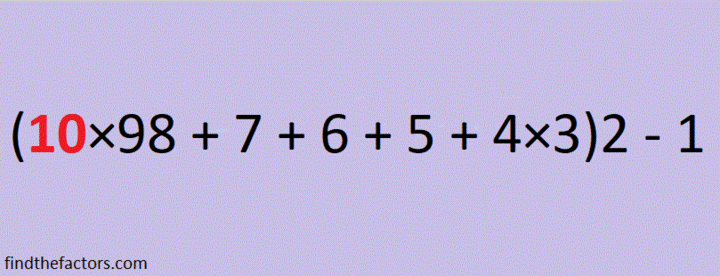make science GIFs like this at MakeaGif

2019 is the sum of consecutive numbers three different ways:
1009 + 1010 = 2019
672 + 673 + 674 = 2019
334 + 335 + 336 + 337 + 338 + 339 = 2019

There is one way that 2019 is the sum of consecutive odd numbers:
671 + 673 + 675 = 2019

2019 is the difference of two squares two different ways:
338² – 335² = 2019
1010² – 1009² = 2019

2019 is the sum of three squares nine different ways:
43² + 13² + 1² = 2019
43² + 11² + 7² = 2019
41² + 17² + 7² = 2019
41² + 13² + 13² = 2019
37² + 25² + 5² = 2019
37² + 23² + 11² = 2019
37² + 19² + 17² = 2019
35² + 25² + 13² = 2019
31² + 23² + 23² = 2019

2019 is the hypotenuse of a Pythagorean triple:
1155-1656-2019 so 1155² + 1656² = 2019²

2¹⁰ + 2⁹ + 2⁸ + 2⁷ + 2⁶ + 2⁵ + 2¹ + 2⁰ = 2019

2019 is a palindrome in a couple of bases:
It’s 5B5 in BASE 19 (B is 11 base 10) because 5(19²) + 11(19) + 5(1) = 2019,
and 3C3 in BASE 24 (C is 12 base 10) because 3(24²) + 12(24) + 3(1) = 2019

Every year has factors that often catch people by surprise. Today I would like to give you my predictions for the factors of 2019:
2019 will have four positive factors: 1, 3, 673, and 2019
However, 2019 will also have four negative factors: -1, -3, -673, and -2019

Which factors, positive or negative, will be your focus in the coming year?Finally, I’ll share some mathematics-related 2019 and New Year tweets that I’ve seen on twitter. Some of these tweets have links that contain even more facts about the number 2019.

That tweet inspired me to make my own 3 × 3 Magic Square where every number is different but every row, column and diagonal totals the same number:
But because 2019 is divisible by 3, it can also be a magic sum of 3 × 3 magic square:5

5

And finally, here is my contribution to 2019 twitter:

# 1192 Experiential Learning Helps Students Understand Their Capacity

Sara Van Der Werf inspired me last year with her post titled Why You Need a PLAY TABLE in your mathematics classroom ASAP. I’ve seen merit in using play to teach and learn mathematics for some time now. What Sara’s post did was make me long to be back in a mathematics classroom so I could have such a play table!

Last week I started working at the American Academy of Innovation, a charter school nearby that engages students in learning through projects and other activities.

Each member of the faculty was given the assignment to make something that reflects his or her teaching philosophy. Here’s the one I put together:On my display, I included the words, “Experiential Learning Helps Students Understand Their Capacity.” That’s my teaching philosophy.

The wording I used was intentional.

•  “Their” might refer to the students or to the geometric solids.
• “Capacity” has two appropriate definitions stated so perfectly by Google in the screenshot below:
1) the ability or power the students “have to do, experience, or understand something”, and 2) the maximum amount the geometric solids can contain.
The scattered rice symbolizes that these learning experiences can sometimes be messy. We will learn more if we aren’t afraid to make mistakes or a little mess.

I am so excited! I’ve taken all my math toys out of storage and will have an ever-changing play table in my classroom where students can play and learn mathematics. Thank you, Sara Van Der Werf for inspiring me!

Now I’ll write a little about the number 1192:

• 1192 is a composite number.
• Prime factorization: 1192 = 2 × 2 × 2 × 149, which can be written 1192 = 2³ × 149
• The exponents in the prime factorization are 3 and 1. Adding one to each and multiplying we get (3 + 1)(1 + 1) = 4 × 2 = 8. Therefore 1192 has exactly 8 factors.
• Factors of 1192: 1, 2, 4, 8, 149, 298, 596, 1192
• Factor pairs: 1192 = 1 × 1192, 2 × 596, 4 × 298, or 8 × 149
• Taking the factor pair with the largest square number factor, we get √1192 = (√4)(√298) = 2√298 ≈ 34.525351192 is the sum of two consecutive prime numbers:
593 + 599 = 1192

34² +  6² = 1192

1192 is the hypotenuse of a Pythagorean triple:
408-1120-1192 calculated from 2(34)( 6), 34² –  6², 34² +  6²
It is also 8 times (51-140-149)

1192 is a cool-looking 3322 in BASE 7

# 896÷8=112. Math Teachers, It’s Carnival Time!

1. Welcome to the 112th Math Education Blog Carnival! There are many fabulous rides at this carnival, and hopefully you won’t get motion sickness on any of them!At this first booth, we have the mystifying number 112. What is amazing about the number 112?

Well, not only is 112² = 2² + 4² + 6² + 7² + 8² + 9² + 11² + 15² + 16² + 17² + 18² + 19² + 24² + 25² + 27² + 29² + 33² + 35² + 37² + 42² + 50², but 112 is also the side length of the SMALLEST square that is composed entirely of smaller distinct sized squares with integer sides. Sources: Squaring a Square and OEIS.org. There are 21 different squares in this square with side length 112. Click on the image below and it will magically become bigger. You can then print it, cut it into pieces to make a puzzle, and take that home as your first carnival prize today.
I don’t know if it is significant, but 112 is 7 × 4², and 112’s square was divided into 3 × 7 different squares . 175 is also such a side length, and 175 is 7 × 5², and 175’s square was divided into 3 × 8 different squares.

Bedtime Math has a quick math activity for kids every evening. Here’s one about adding, subtracting, and/or multiplying at the Giraffe Hotel.

Algorithms

Rodi Steinig leads a math circle of students ages 11-17 in a course titled “Our Algorithmic Culture,”. It may surprise you that algorithms are not just for math; they are for real, real life, too. See for yourself as several activities are described in Introducing Algorithms, a post about the first of 8 sessions on the subject.

Math Art

RobertLovesPi’s blog regularly features a rotating solid geometric shape or a beautiful tessellation such as this one that can be enjoyed by young or old alike.

David Mitchell of Latticelabyrinths explains how he and his friend, Jacob, made a beautiful structure for the September 1917 Wirksworth Art and Architecture Trail using a large peg board, pegs and 1302 red or blue precisely-cut wooden equilateral triangles. Amazing! I wish I could have seen it in person.

What is a Rotogon? Katie Steckles of aperiodical.com blogged about this beautiful computer generated, constantly transforming piece of art that could mesmerize young and old alike.

Bell Ringers

What is the Same? What is Different?  has a wide variety of thoughtful activities that can get your students’ brain juices flowing.

Try Math Visuals for other great bell ringers.

Life Through a Mathematician’s Eyes sees a great deal of beauty in Pascal’s Triangle, but it isn’t the significant part of the curriculum she wishes it could be. She got around that though. In her post, Pascal’s Triangle, she shared some great articles that she turned into bell ringers for her students to contemplate when they arrive to class. She also made assignments that can be completed in class or at home.

Bulletin Boards

Paul Murray creates bulletin boards near the lunch room with questions on them.  Some of the questions are math problems. For the last ten years or so, students have stopped by his bulletin boards, read them, and pondered the questions. Read how he does it in A Math Bulletin Board that Actually Gets Read!

Carnival of Mathematics

Earlier this month Just Maths published the more advanced 149th Carnival of Mathematics. It has several great links in it that could pique high schoolers’ interest even if those students aren’t able to understand all the mathematics yet. Next month Alexander at CoDiMa will host the 150th Carnival of Mathematics.

Math Competitions

Some people like entering math competitions. If you have a student that likes them, look at this post from Resourceaholic. It has questions that can be a fun challenge whether you like competitions or not.

Games

Alan Parr often plays his envelop game with students who are learning many different mathematical concepts. His students all enjoy it. The game described in A Wow! Conversation with Amy was easy to put together, let Amy display some brilliant reasoning and provided its creator a very memorable experience, perhaps his most memorable this school year. .

Geometry

Paula Beardell Krieg of Bookzoompa’s wrote a post about Symmetry for 4 – 5 year olds that I adore. Even kids that young can make some gorgeous geometric art.

Mike’s Math Page does so many great math videos with his sons that its difficult to pick only one or two. I went with a couple of posts about geometry: Playing with Some Mathy Art ideas this morning which will appeal to kids of all ages and Lessons from a great geometry homework problem for older kids.

Before teaching congruent triangle proofs, Mrs. E Teaches Math recommends a paper folding, cutting, and arranging activity that helps students visualize overlapping triangles.

Mathematical Humor:

A little mathematical humor can help students access prior knowledge, or it can make a new concept memorable. Joseph Nebus reads lots of comics to find ones with some mathematics in them. This August 17, 2017 edition of Reading the Comics has a few that could help students remember what irrational means, what sum means, or what the < sign means.

Math Literature Books

Math Book Magic introduces us to a new book, Stack the Cats by Susie Ghahremani. The book is just right for kindergarten and earlier elementary grades. It can help you talk to kids about counting, adding, even decomposing numbers in a very fun way. Kids may not even be aware they are learning math as they find different ways to stack toy cats and other objects. Stack the Cats joins the #Mathbookmagic

Ben Orlin of Math with Bad Drawings delights us with Literature’s Greatest Opening Lines, as Written By Mathematicians.

Denise Gaskins has written Word Problems from Literature. It has a more serious but still very fun approach to exploring mathematics and problem solving through good literature.

Math Magic

Alex Bellos of Science Book a Day introduces us to Magical Mathematics: The Mathematical Ideas That Animate Great Magic Tricks. Every trick in the book introduces a different mathematical idea, and lots of magician secrets are revealed. Sounds fun!

Managing the Mathematics Classroom

Mrs. G. of Give Me a Sine blogged that this school year she had the best first week of school she’s ever had. She mixed some of her tried and true favorite activities with some new ones that she learned this summer from NYC Math Lab and Sara Vanderwerf. Mrs. G gives specifics detailing what she did. Her students’ response described in the next to the last paragraph is enviable.

Years ago I made a seating chart for a Pre-Algebra class. I didn’t know the students yet and arranged my seating chart in alphabetical order by first name. It was a disaster. I had placed the tallest student in the class at a desk in front of the shortest student in the class. Once one legitimate complaint was voiced, other complaints followed. If I could have read Mrs. E Teaches Math post How to Create a Seating Chart before that school year, I would have had one less frustration.

Math Music

Coleen Young has updated the Mathematical Songs on her website to include MinuteMath’s quadratic formula sung to a One Direction song. She wrote that the song makes her students smile and sing along.

Number Sense

What’s your favorite number? John Golden of Math Hombre asked that question to people and got some wonderful responses. Really cool mathematics is attached to several numbers including the Top Ten Favorite Numbers.

Problem Solving

True problem solving is more about “Why?” than it is about an exact “What?” Denise Gaskins of Let’s Play Math has written several questions to teach students How to Succeed in Math: Answer-Getting vs. Problem-Solving

Puzzles

Long ago mathematicians were often philosophers and philosophers were often mathematicians. Simona Prilogan is both. Every day she posts a mathematical meme, a puzzle such as this one, and a philosophical mathematical thought. Search her site for all kinds of goodies to appeal mostly to middle school children and up.

Alok Goyal’s Puzzles Page shared a puzzle titled 10 Friends. Upper elementary students will be able to understand what the puzzle is asking but would probably need a lot of guidance to solve it.

Rupesh Gesota of Math Coach shared an interesting 6 rectangle puzzle and revealed several different methods students used to solve the puzzle.

My blog typically features a factoring puzzle such as this one that I fancied up for back to school:This month I did something different: I wrote an elementary-school-age time management lesson plan with an object lesson that uses Tangram puzzle pieces. It was a big hit with the teachers and the students.

Resources

Three J’s Learning wrote math recommendations for 3 year-olds! It includes a list of measuring devices a 3 year-old would love to use and learn from.

Singapore Maths Tuition has assembled a list of the Best Online Resources to Improve Your Math Skills along with their pros and cons.

Resource Room Dot Net Blog wrote about an experience using Illustrative Mathematics (a resource for 6-8 grades) and how it is worth the effort to give feedback.

Square Roots

Last month’s 111 Math Teachers at Play Carnival was hosted at High Heels and No. 2 Pencils by Jacqueline Richardson. This month the Amazing Jacqueline doesn’t just guess your age, she can guess the square root of your age within a few decimal places! I have never seen anything like this before. She uses tiles and grid paper to model square roots of non-perfect squares and is amazingly accurate. Teach your students this way, and they will be amazed. They will understand square roots so well that they will be amazing as well.

Teaching Practices

What are the zeros of this polynomial? Julie Morgan of Fraction Fanatic lets her students give their solutions in a colorful way. This practice has become her new favorite.  Lots of discussion happens. Young children could also use this fun method to give answers.

Ed Southall of Solvemymaths wrote a sobering post titled Sorry we keep lying to you… about lies our math teachers told us and we continue to perpetuate. Read it. Share it. Maybe the lies will stop.

Sara Vanderwerf explains how her Stand and Talks engage students much more than Think/Pair/Share does and REALLY gets the whole class talking about math and contributing to a whole class discussion.

Statitistics

Business blogger Lanisha Butterfield wrote a fascinating article titled Arithmophobia. A major portion of the article was an interview with statistician Jennifer Rogers who did well in math as a kid but HATED it until she was introduced to A-level maths and statistics in school. High School teachers and students should be especially interested in this article.

Sue VanHattum of Math Mama Writes detailed her first day teaching algebra, statistics, and calculus this year. When she discussed the class syllabus, she inserted some fun mathematics here and there. She summed up that first day and shared every math teacher’s universal dream, “I think the class went well. If they really feel good about it, they’ll end up thinking I’m their best teacher ever.”

To everyone who plays at this Carnival, I hope your students think that way about you!

Thank you to everyone who blogged about teaching mathematics this month, and especially thank you to those who submitted a post to this carnival.

I’d like to encourage everyone who blogs about math to submit a post to next month’s carnival which will be hosted at Three J’s Learning.

***************

Since this is my 896th post, I’ll also write a little bit about that number which happens to be 8 times 112. A factor tree for 112 is contained in this factor tree for 896.896 is SS in BASE 31 (S is 28 base 10), because 28(31) + 28(1) = 28(31 + 1) = 28(32) = 896.

896 is also S0 in BASE 32 because 28(32) + 0(1) = 896.

896 is the sum of six consecutive prime numbers: 137 + 139 + 149 + 151 + 157 + 163 = 896.

Here is the factoring information for 896 with the ten factors of 112 in red.

• 896 is a composite number.
• Prime factorization: 896 = 2 × 2 × 2 × 2 × 2 × 2 × 2 × 7, which can be written 896 = 2⁽⁴⁺³ × 7 or 896 = 2⁷ × 7
• The exponents in the prime factorization are 7 and 1. Adding one to each and multiplying we get (7 + 1)(1 + 1) = 8 × 2 = 16. Therefore 896 has exactly 16 factors.
• Factors of 896: 12, 4, 7, 8, 14, 16, 28, 32, 56, 64, 112, 128, 224, 448, 896
• Factor pairs: 896 = 1 × 896, 2 × 448, 4 × 224, 7 × 128, 8 × 112, 14 × 64, 16 × 56, or 28 × 32
• Taking the factor pair with the largest square number factor, we get √896 = (√64)(√14) = 8√14 ≈ 29.933259
# 837 and Level 3

837 is repdigit RR in BASE 30 (R is 27 in base 10). All that means is that 27(30¹) + 27(30º) = 837, which naturally follows from the fact that 27(30 + 1) = 837.

837 has four odd factor pairs, so 837 can be written as the difference of two squares four different ways:

• 837 × 1 = 837 means 419² – 418² = 837
• 279 × 3 = 837 means 141² – 138² = 837
• 93 × 9 = 837 means 51² – 42² = 837
• 31 × 27 = 837 means 29² – 2² = 837

Hmm…837 is only four numbers away from the next perfect square, 841.Print the puzzles or type the solution on this excel file: 10-factors-835-842

• 837 is a composite number.
• Prime factorization: 837 = 3 × 3 × 3 × 31, which can be written 837 = 3³ × 31
• The exponents in the prime factorization are 3 and 1. Adding one to each and multiplying we get (3 + 1)(1 + 1) = 4 × 2 = 8. Therefore 837 has exactly 8 factors.
• Factors of 837: 1, 3, 9, 27, 31, 93, 279, 837
• Factor pairs: 837 = 1 × 837, 3 × 279, 9 × 93, or 27 × 31
• Taking the factor pair with the largest square number factor, we get √837 = (√9)(√93) = 3√93 ≈ 28.93095
# 834 and Level 6834 is the sum of consecutive prime numbers two different ways:

• 127 + 131 + 137 + 139 + 149 + 151 = 834; that’s six consecutive primes
• 31 + 37 + 41 + 43 + 47 + 53 + 59 + 61 + 67 + 71 + 73 + 79 + 83 + 89 = 834; that’s fourteen consecutive primes

The ONLY Pythagorean triple that contains the number 834 is 834 – 173888 – 173890.

• 834 is a composite number.
• Prime factorization: 834 = 2 × 3 × 139
• The exponents in the prime factorization are 1, 1, and 1. Adding one to each and multiplying we get (1 + 1)(1 + 1)(1 + 1) = 2 × 2 × 2 = 8. Therefore 834 has exactly 8 factors.
• Factors of 834: 1, 2, 3, 6, 139, 278, 417, 834
• Factor pairs: 834 = 1 × 834, 2 × 417, 3 × 278, or 6 × 139
• 834 has no square factors that allow its square root to be simplified. √834 ≈ 28.879058There was a solar eclipse in the United States today. People where I lived were able to experience 91.32% obstruction of the sun. I love this interactive map of today’s eclipse and past and future ones as well.

Several people have taken and shared marvelous pictures of the eclipse.# 833 and Level 5Print the puzzles or type the solution on this excel file: 12 factors 829-834

Wrap Your Mind Around This:  × 17 = 833 = 28² + 7²

833 is the hypotenuse of a Pythagorean triple:

• 392-735-833 calculated from 2(28)(7), 28² – 7², 28² + 7²

833 can be written as the sum of consecutive prime numbers two ways. One of those ways starts with one of its prime factors, 17:

• 833 is the sum of the seventeen prime numbers from 17 to 83
• 833 is also the sum of the eleven prime numbers from 53 to 101

Since 833 has three factor pairs where both factors are odd, it can be written as the difference of two squares three different ways:

• 833 × 1 = 833 means 417² – 416² = 833
• 119 × 7 = 833 means 63² – 56² = 833
• 49 × 17 = 833 means 33² – 16² = 833

The 41st triangular number will be 861. We must use less than 41 consecutive numbers if we want to express 833 as the sum of consecutive numbers. 833 has 3 odd factors (1, 7, 17) less than 41. Thus 833 can be written as the sum of 7 consecutive numbers and as the sum of 17 consecutive numbers. Notice 833’s factor pairs below highlighted in red.

• 833 = 116 + 117 + 118 + 119 + 120 + 121 + 122; that’s 7 consecutive numbers
• 833 = 41 + 42 + 43 + 44 + 45 + 46 + 47 + 48 + 49 + 50 + 51 + 52 + 53 + 54 + 55 + 56 + 57; that’s 17 consecutive numbers

The factor of 833 that is the highest power of 2 is 1 because 2º=1. Each of those odd factors, (1, 7, 17), times 2 × 1 is still less than 40, so 833 can also be written as the sum of 2 consecutive numbers, the sum of 14 consecutive numbers, and the sum of 34 consecutive numbers:

• 833 = 416 + 417; that’s 2 consecutive numbers
• 833 = 53 + 54 + 55 + 56 + 57 + 58 + 59 + 60 + 61 + 62 + 63 + 64 + 65 + 66; that’s 14 consecutive numbers
• 833 = 8 + 9 + 10 + 11 + 12 + 13 + 14 + 15 + 16 + 17 + 18 + 19 + 20 + 21 + 22 + 23 + 24 + 25 + 26 + 27 + 28 + 29 + 30 + 31 + 32 + 33 + 34 + 35 + 36 + 37 + 38 + 39 + 40 + 41; that’s 34 consecutive numbers

Here is 833’s factoring information:

• 833 is a composite number.
• Prime factorization: 833 = 7 × 7 × 17, which can be written 833 = 7² × 17
• The exponents in the prime factorization are 2 and 1. Adding one to each and multiplying we get (2 + 1)(1 + 1) = 3 × 2  = 6. Therefore 833 has exactly 6 factors.
• Factors of 833: 1, 7, 17, 49, 119, 833
• Factor pairs: 833 = 1 × 833, 7 × 119, or 17 × 49
• Taking the factor pair with the largest square number factor, we get √833 = (√49)(√17) = 7√17 ≈ 28.861739
# 832 and Level 4

832 has many factors, but it can be written as the sum of consecutive numbers only one way:

• 58 + 59 + 60 + 61 + 62 + 63 + 64 + 65 + 66 + 67 + 68 + 69 + 70 = 832; that’s thirteen consecutive numbers.

832 can be written as the difference of two squares five different ways because it has five factor pairs in which both numbers are even:

• 416 × 2 = 832 means 209² – 207² = 832
• 208 × 4 = 832 means 106² – 102² = 832
• 104 × 8 = 832 means 56² – 48² = 832
• 52 × 16 = 832 means 34² – 18² = 832
• 32 × 26 = 832 means 29² – 3² = 832

832 is also the sum of two squares:

• 24² + 16² = 832

832 is the hypotenuse of a Pythagorean triple:

• 320-768-832 calculated from 24² – 16², 2(24)(16), 24² + 16²
• 320-768-832 is also 64 times (5-12-13)

832 is repdigit QQ in BASE 31 (Q is 26 base 10). That’s because 26(31) + 26(1) = 832, which is the same as saying 26 × 32 = 832.

• 832 is a composite number.
• Prime factorization: 832 = 2 × 2 × 2 × 2 × 2 × 2 × 13, which can be written 832 = 2⁶ × 13
• The exponents in the prime factorization are 6, and 1. Adding one to each and multiplying we get (6 + 1)(1 + 1) = 7 × 2 = 14. Therefore 832 has exactly 14 factors.
• Factors of 832: 1, 2, 4, 8, 13, 16, 26, 32, 52, 64, 104, 208, 416, 832
• Factor pairs: 832 = 1 × 832, 2 × 416, 4 × 208, 8 × 104, 13 × 64, 16 × 52, or 26 × 32
• Taking the factor pair with the largest square number factor, we get √832 = (√64)(√13) = 8√13 ≈ 28.8444102